Function Repository Resource:

# MeanValueTheoremQuiz

Generate a quiz to assess understanding of the mean value theorem for derivatives

Contributed by: Wolfram|Alpha Math Team
 ResourceFunction["MeanValueTheoremQuiz"][] returns an assessment on visualizing the mean value theorem (MVT) for derivatives.

## Details

ResourceFunction["MeanValueTheoremQuiz"][] randomly picks a function and interval on the x axis. The user is asked to verify if the conditions of the MVT hold for the given function on the given interval and then plot the line tangent to the curve and parallel to the secant line of the curve between the endpoints of the interval.
For each problem, students must first use the drop-down menu to indicate whether the conditions of the MVT are met (i.e. the curve is continuous and differentiable on the given interval). Some functions will be noncontinuous or nondifferentiable.
The interface returned by ResourceFunction["MeanValueTheoremQuiz"] includes the following buttons:
 Check answer automatically grades input and gives feedback Reset problem resets the problem by removing user-chosen points Next problem randomly generates a new problem and internally saves the previous problem history Print report prints a description of the problems that have been attempted so far, all guesses made and whether the last guess on each problem was correct
Styling and interface controls may be found under the "Display options" opener.
ResourceFunction["MeanValueTheoremQuiz"] accepts the option "TangentPoint" (default False) that determines whether to include a checkbox under the "Display options" opener to show the correct point(s) of tangency.

## Examples

### Basic Examples (1)

Generate an interface to quiz yourself on visualizing the mean value theorem (MVT) for a function on a given interval:

 In:=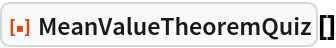Out=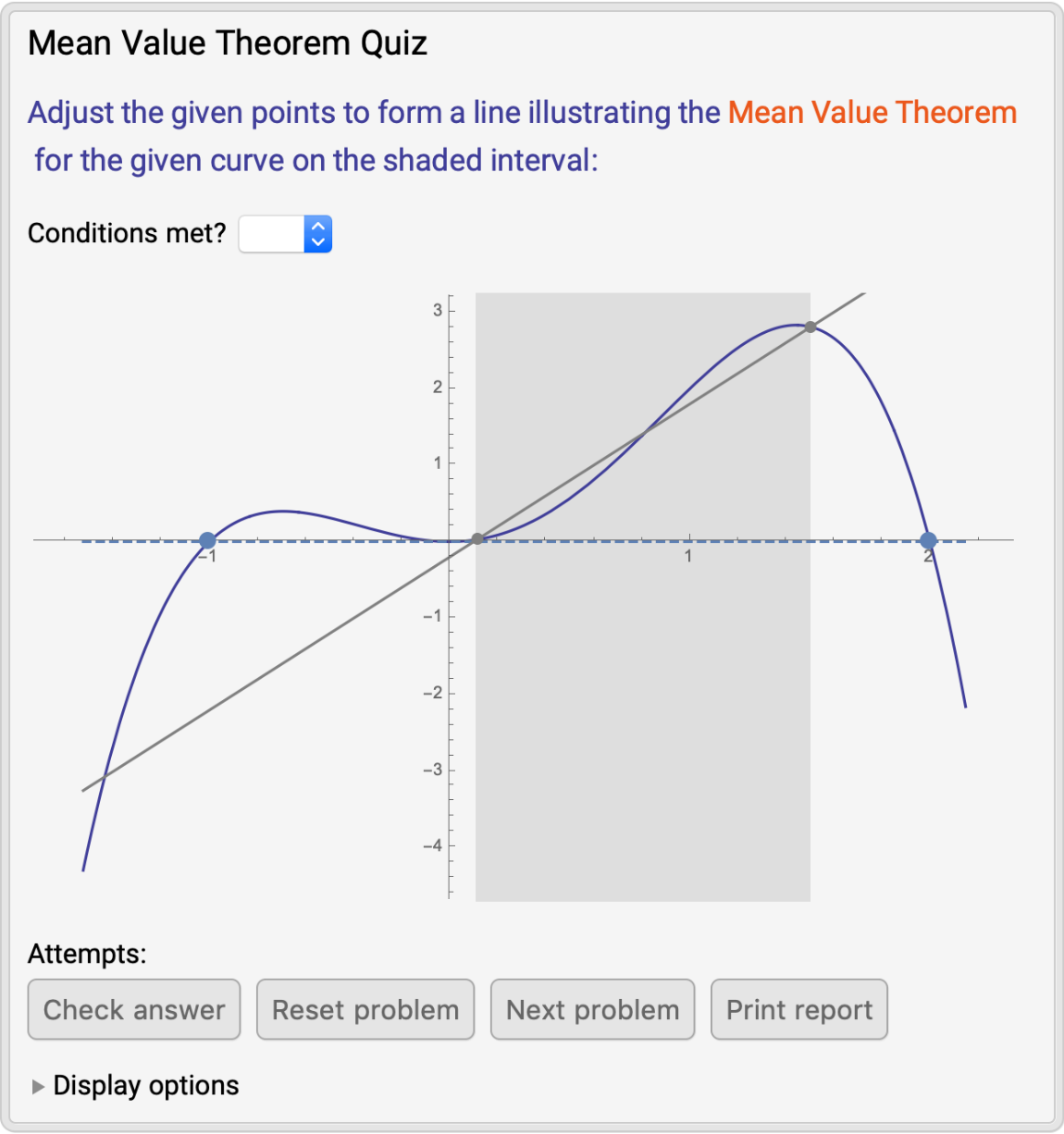### Scope (6)

When the Check answer button is clicked, the latest attempt is marked based on correct verification of the conditions for the MVT and approximately correct placement of a relevant tangent line. Correct attempts receive a green check mark:

 In:=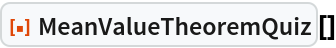Out=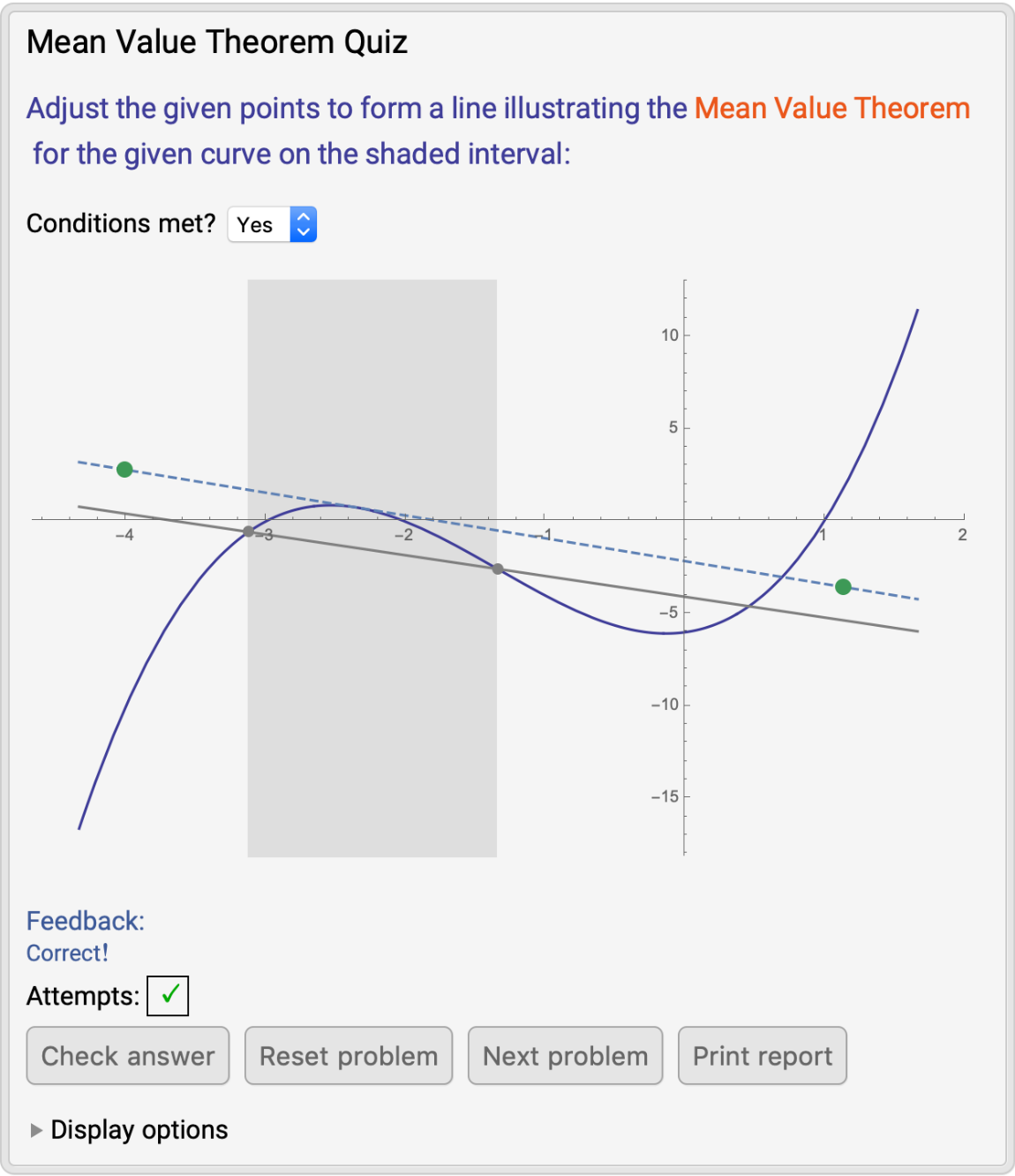In the case that there are multiple tangent lines that satisfy the conclusion of the MVT, any of those lines is graded as correct:

 In:=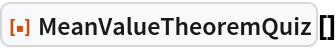Out=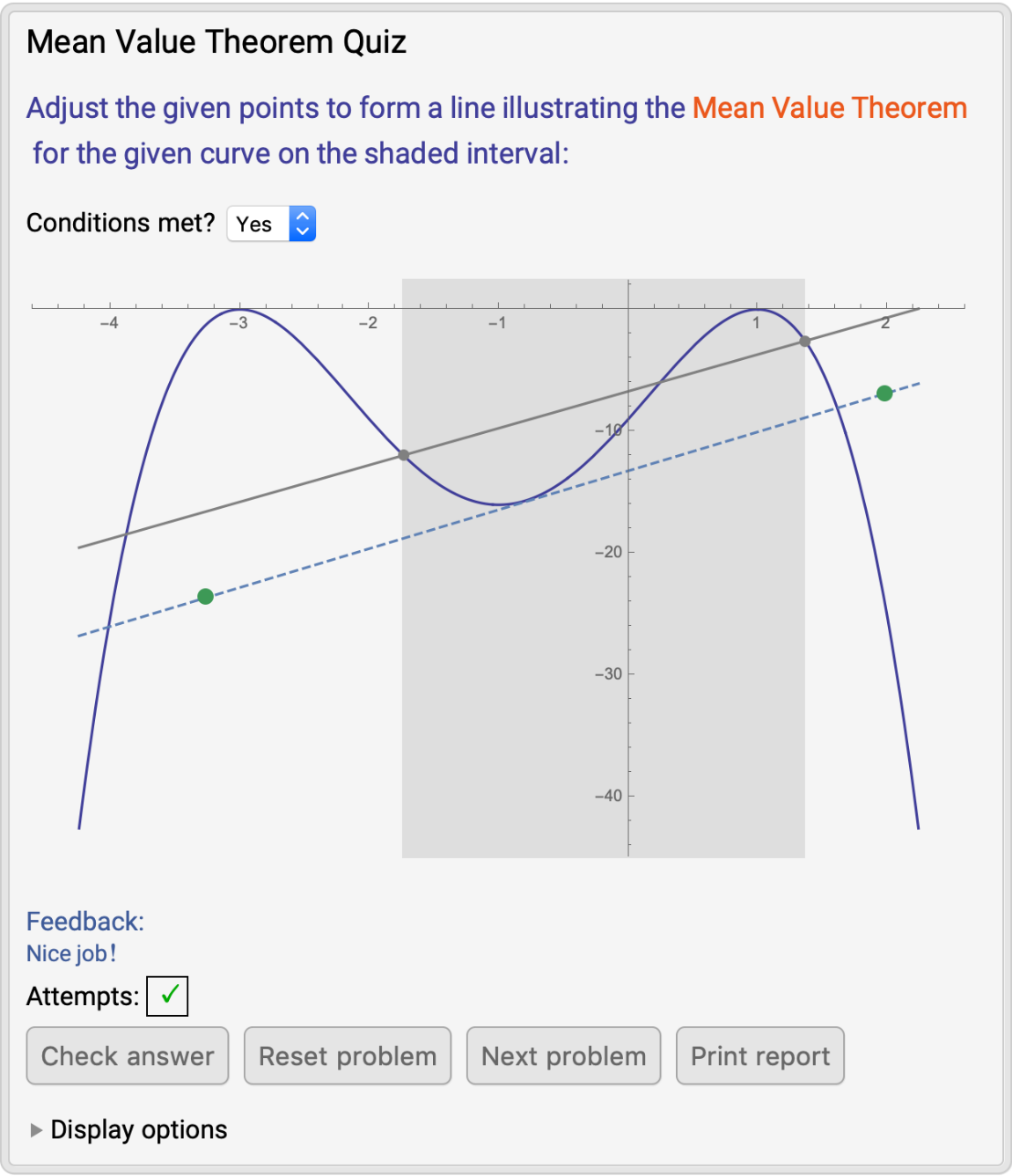If an attempt does not approximately meet the specified criteria, it is marked as incorrect with a red x and the student receives feedback to guide their next attempt:

 In:=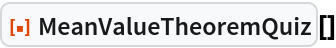Out=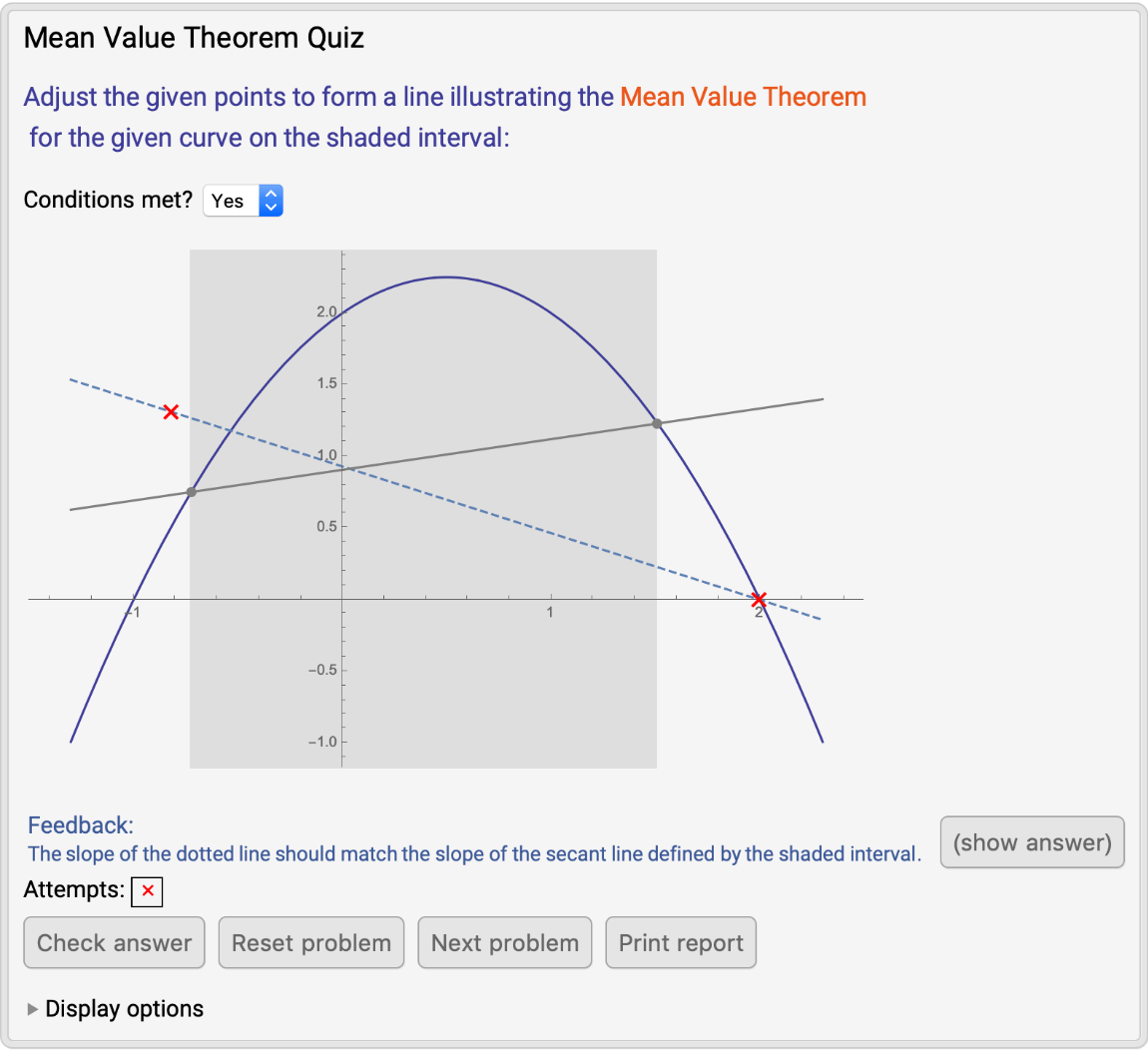Clicking the (show answer) button prints the correct tangent line(s) and prohibits students from submitting any further answers to the current problem:

 In:=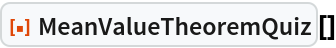Out=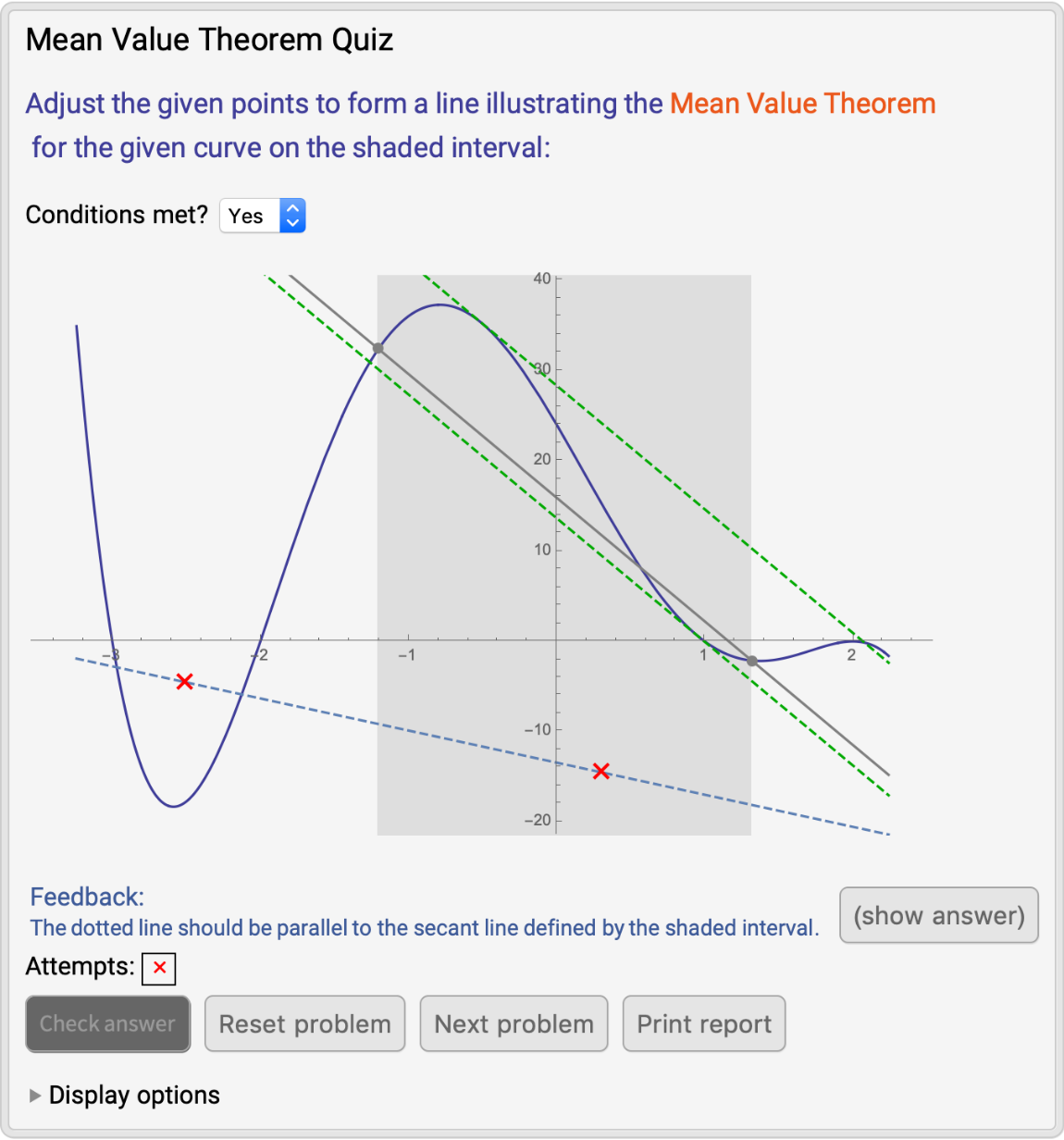Sometimes, the given function will not satisfy the conditions of the MVT. As long as this is indicated accordingly in the drop-down menu, the placement of the locators will not affect the correctness of the attempt:

 In:=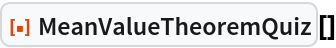Out=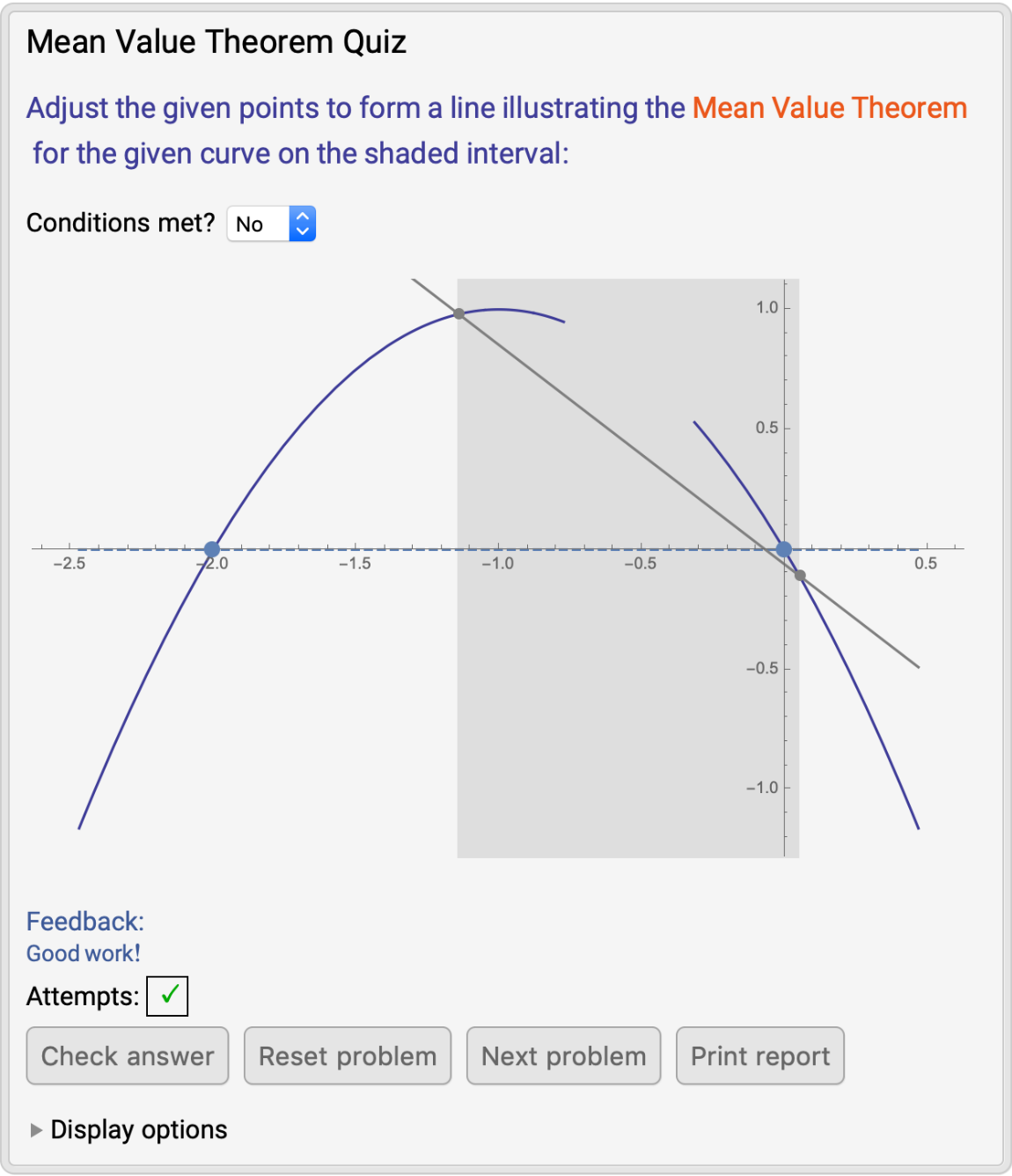Clicking the Print report button returns a description of the problems that have been attempted so far, all guesses made and whether the last guess on each problem was correct:

 In:=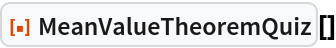Out=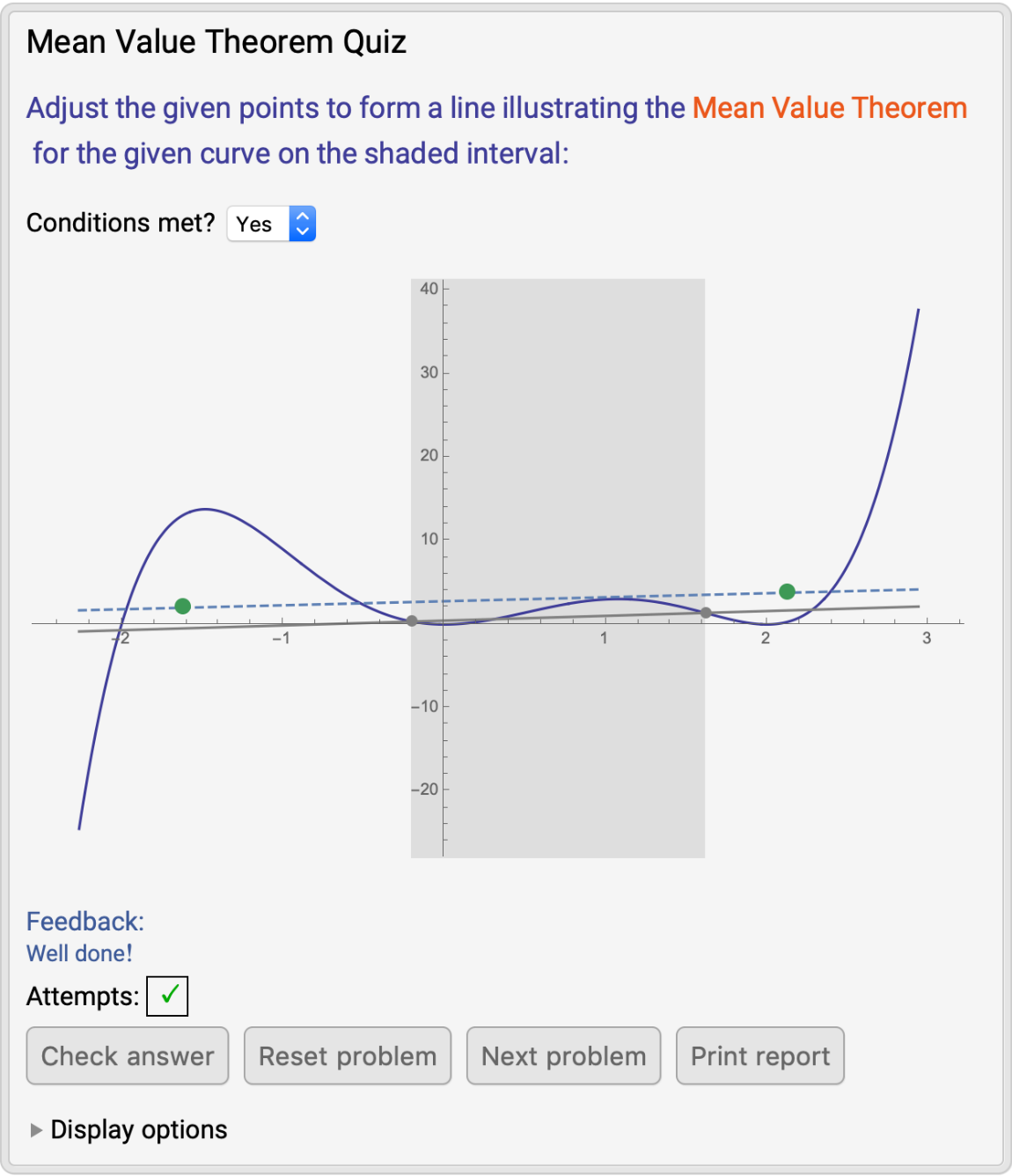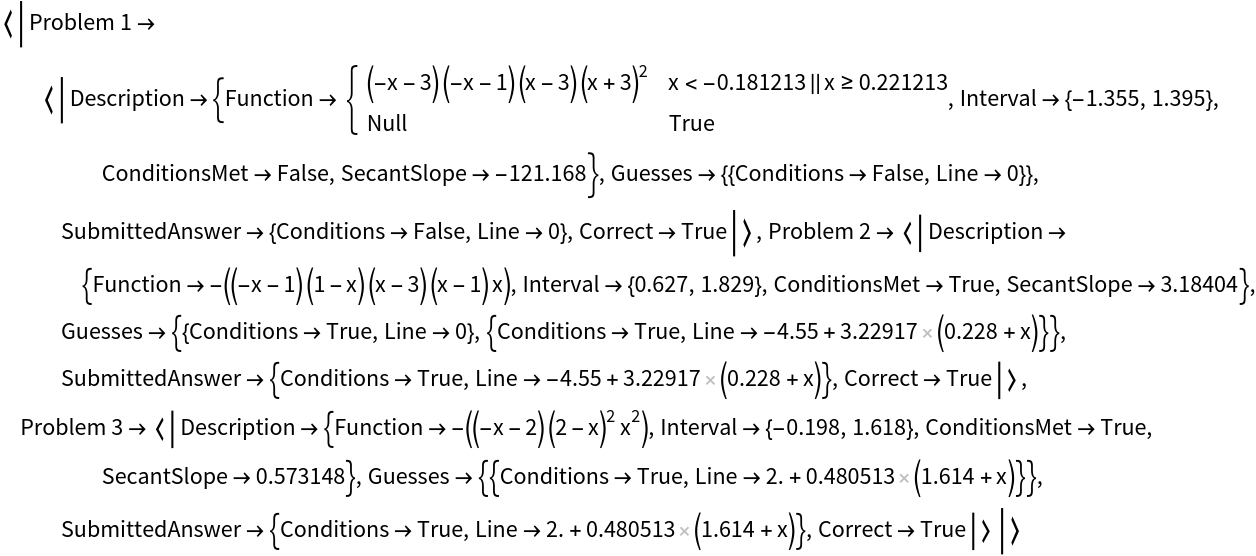### Options (2)

Add a checkbox to the "Display options" opener to control display of the point(s) indicating where the dotted line should be tangent to the curve:

 In:=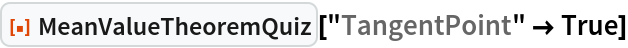Out=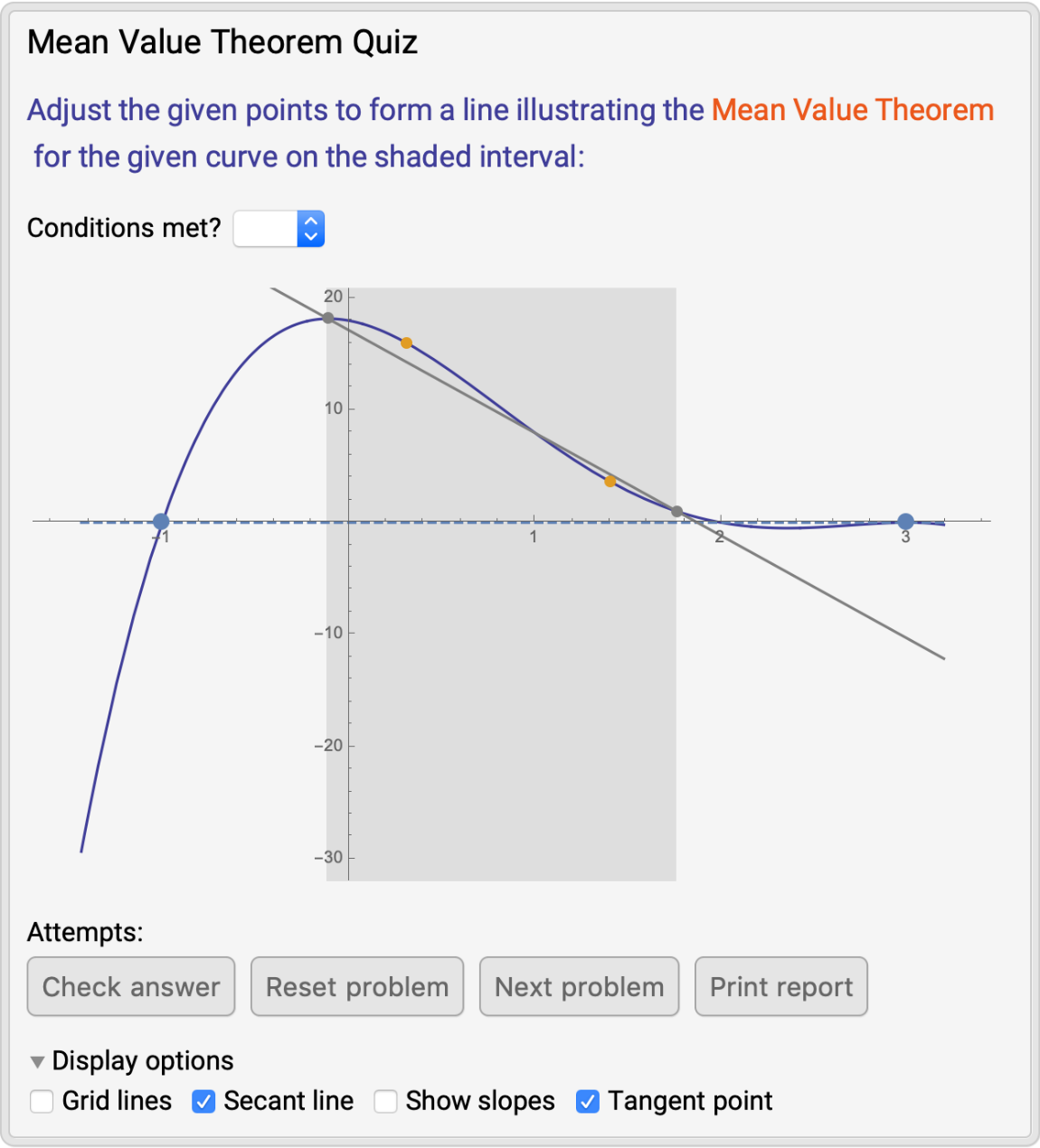Without a "Tangent point" checkbox:

 In:=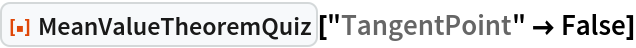Out=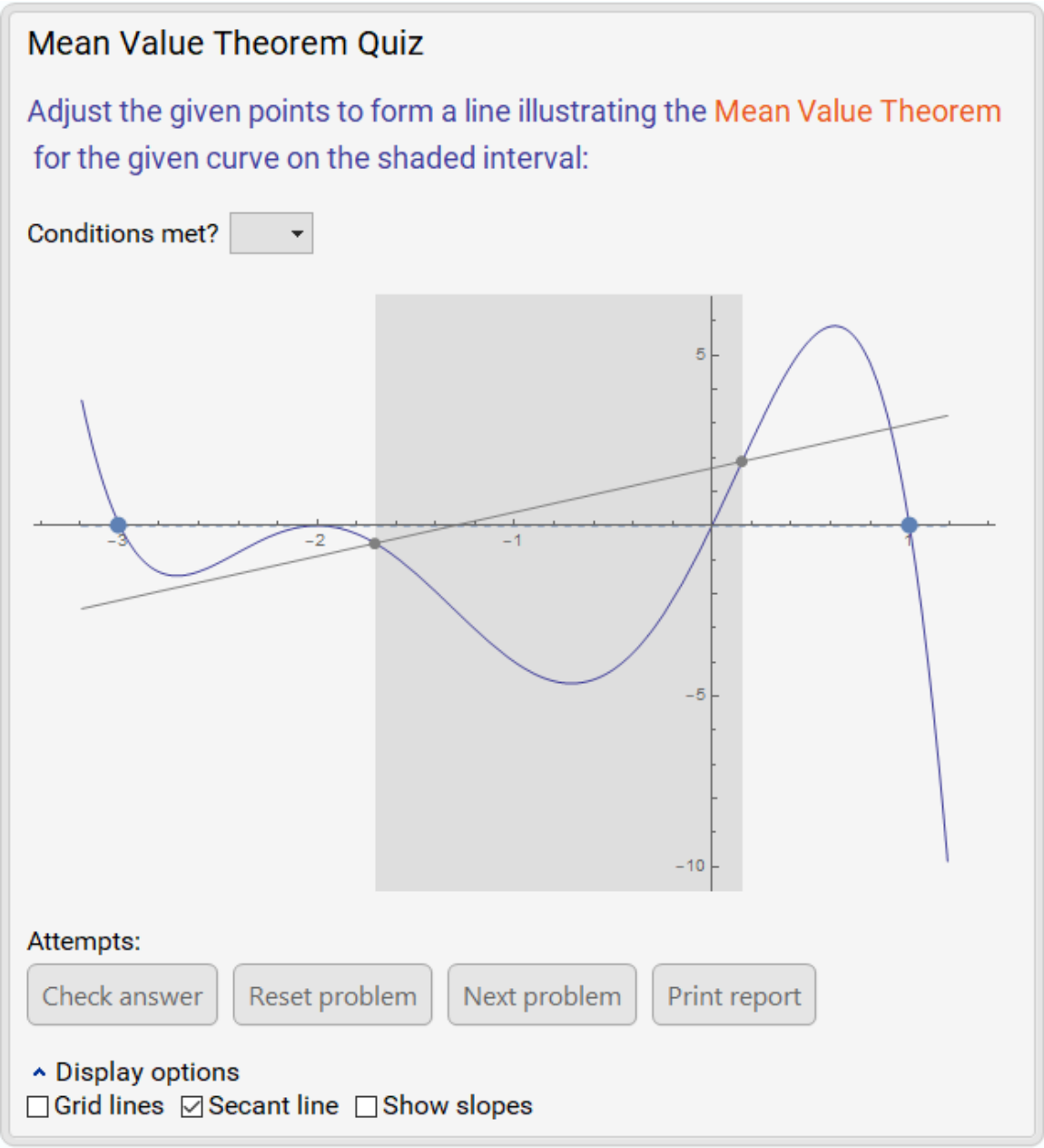## Publisher

Wolfram|Alpha Math Team

## Version History

• 2.0.0 – 23 March 2023
• 1.0.0 – 23 February 2021

## Author Notes

To view the full source code for MeanValueTheoremQuiz, evaluate the following: